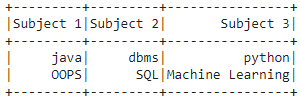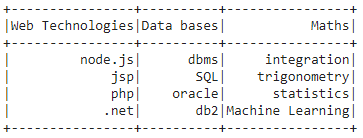# PySpark – Create DataFrame from List

• Last Updated : 30 May, 2021

In this article, we are going to discuss how to create a Pyspark dataframe from a list.

To do this first create a list of data and a list of column names. Then pass this zipped data to spark.createDataFrame() method. This method is used to create DataFrame. The data attribute will be the list of data and the columns attribute will be the list of names.

dataframe = spark.createDataFrame(data, columns)

Example1: Python code to create Pyspark student dataframe from two lists.

## Python3

 `# importing module``import` `pyspark`` ` `# importing sparksession from ``# pyspark.sql module``from` `pyspark.sql ``import` `SparkSession`` ` `# creating sparksession and giving ``# an app name``spark ``=` `SparkSession.builder.appName(``'sparkdf'``).getOrCreate()`` ` `# list  of college data with two lists``data ``=` `[[``"java"``, ``"dbms"``, ``"python"``], ``        ``[``"OOPS"``, ``"SQL"``, ``"Machine Learning"``]]`` ` `# giving column names of dataframe``columns ``=` `[``"Subject 1"``, ``"Subject 2"``, ``"Subject 3"``]`` ` `# creating a dataframe``dataframe ``=` `spark.createDataFrame(data, columns)`` ` `# show data frame``dataframe.show()`

Output:Example 2: Create a dataframe from 4 lists

## Python3

 `# importing module``import` `pyspark`` ` `# importing sparksession from ``# pyspark.sql module``from` `pyspark.sql ``import` `SparkSession`` ` `# creating sparksession and giving ``# an app name``spark ``=` `SparkSession.builder.appName(``'sparkdf'``).getOrCreate()`` ` `# list  of college data with two lists``data ``=` `[[``"node.js"``, ``"dbms"``, ``"integration"``],``        ``[``"jsp"``, ``"SQL"``, ``"trigonometry"``],``        ``[``"php"``, ``"oracle"``, ``"statistics"``],``        ``[``".net"``, ``"db2"``, ``"Machine Learning"``]]`` ` `# giving column names of dataframe``columns ``=` `[``"Web Technologies"``, ``"Data bases"``, ``"Maths"``]`` ` `# creating a dataframe``dataframe ``=` `spark.createDataFrame(data, columns)`` ` `# show data frame``dataframe.show()`

Output:My Personal Notes arrow_drop_up